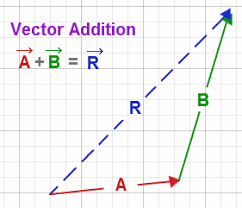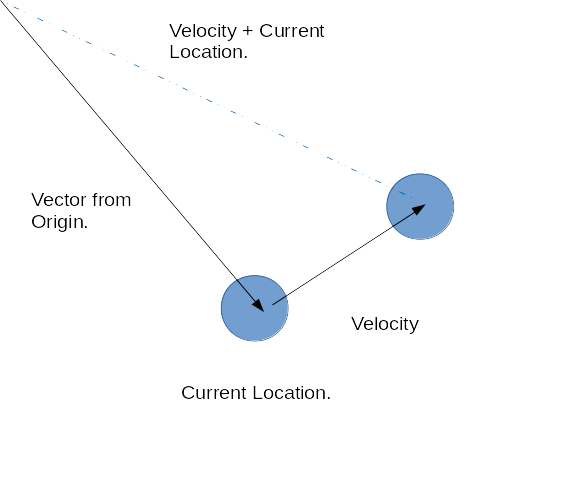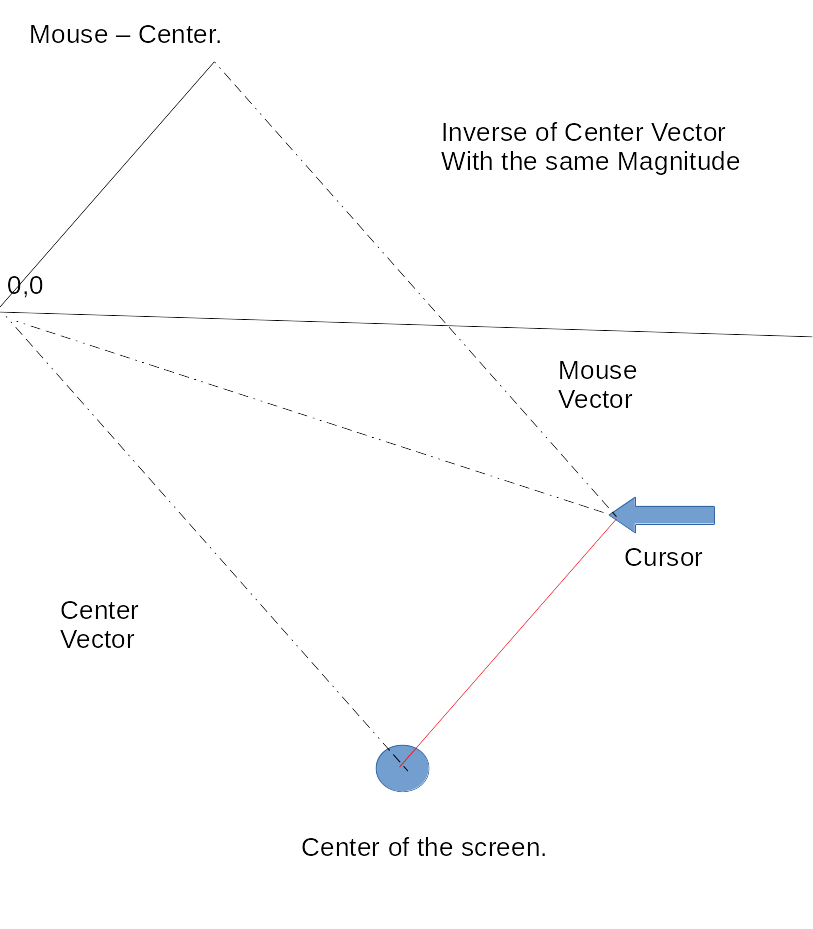24 January 2018, 18:08

# more vectors

As expected my schedule is only getting busier, however I have been squeezing in some learning on my lunch breaks. I learnt a little more about vectors and vector math this week so I'm sharing my notes.

Last post I looked at add a vectors together to vectors, let's remind ourselves how that works:In the above diagram we are adding A to B to get R. We are putting these vectors A and B end to end to get R. This new line R is the result of adding the two.

This maps to the idea of location and velocity, let's draw out our bouncing ball example:Here is the example in code:

See the Pen Simple Bouncing Ball Vector by Adam Harpur (@harps116) on CodePen.

We add the location to velocity to get the new location. The formula in psuedo-code:

``````newLocation.x = location.x + velocity.x
newLocation.y = location.y + velocity.y

``````

Now that we have have a handle on adding let's look at subtraction. There isn't that much difference. All we are doing is replacing the add with subtract but it does require a little extra thinking. Lets first write the pseudo code and then draw it out.

``````W.x = V.x - U.x
W.y = V.y - U.y

``````

We'll be able to give these letters more meaning after we draw it out:To subtract the vectors we lay the vectors end to end but when subtracting we inverse the direction of one keeping the magnitude ( size ). The vector between the two is the result. In this case we have found the Velocity between the two points.

now in we can label those letters:

``````velocity.x = mouseV.x - centerV.x
velocity.y = mouseV.y - centerV.y

``````

Here's an example using code:

See the Pen Vector Subtraction P5 by Adam Harpur (@harps116) on CodePen.

It is best to follow the link to see the full functionality, hover your mouse around the screen and the line will follow.

That's all I managed this week.